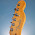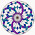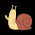## Tuesday, July 15, 2014

### The 216 Golden Rectangle

Nikola Tesla had said quite enigmatically, “If you only knew the magnificence of the 3, 6 and 9, then you would have a key to the universe.”

I think I found the key.  It is like a cipher.  It is very similar to the Vedic Square.  I had no idea of the Vedic Square existed until I looked online to see if anyone else had found this pattern I found.

Fibonacci sequence is an approximation of the Golden Ratio.  The Golden Ratio is within all of nature.

Long story short, I started playing around with Fibonacci.  I thought, What if I start Fibonacci sequence with other numbers besides 1?

Fibonacci sequence is simple.  You start with 1 and 1, and add together.  2.  You take the sum you just acquired (2), and add it to the number right before it.  2+1 = 3.  Then, 3+2 = 5.

The string of sums ends up looking like this: 1, 2, 3, 5, 8, 13, 21, 34, 55, and on and on.  See more here if you want.  [link to en.wikipedia.org] It ends up creating a spiral, called the Golden Spiral.  It is seen in most examples with the Nautilus Shell.

I decided to see what would happen if I started Fibonacci on the 9 single digit numbers (1-9).  When I put the results in graph form, the lines were essentially all the same, but with very cool 2-D wave like form in the graph from the 9 different Fibonacci starting numbers and their solutions.

Then, I decided to take all the solutions and reduce them to single digit numbers.  I found that the solutions repeated themselves.  I didn’t expect this at all, because the Golden Ration does not have a pattern to it.  The decimal string just goes on forever without any kind of discernible pattern.  When the solutions are reduced to single digit, all the numbers 1-9 and their solutions had repeating properties.

If you start Fibonacci sequence on the number 1, and reduce the sums to single digit numbers, it creates a repeating pattern of seemingly random/no pattern single digits.  This repeats every 24 steps of doing Fibonacci on the number 1.

I started Fib on number 2 instead of 1, and did the same thing, and had the same resulting pattern of seemingly random numbers that repeat every 24 steps of Fib.

Then, when I started Fib with the number 3, I found that the Fib solutions when reduced to single digit numbers, repeated every 8th step, not every 24th like numbers 1 and 2.

So, if you start the Fibonacci sequence with numbers 1, 2, 4, 5, 7, or 8, the solutions have a seemingly random string of numbers that repeat exactly every 24th step.  Numbers 3 and 6 repeat a seemingly random string of numbers every 8th step.  Number 9 repeats the solution of 9 infinitely.

The ONLY variation I found in this is that the second string of solutions, which is steps 25-48 of Fibonacci, was that there was a sum of 2 where it should have been 1.  Other than that, no matter how far you go doing Fibonacci and reducing the solution to single digit, it always repeats the exact same string of numbers every 24th steps of Fib.

This results in a table of numbers that is absolutely incredible in the way it creates relationships and patterns.

The Magic Square is a similar type of table of numbers that has unique properties.Then, there is the Vedic Square.  I didn’t even know the Vedic Square existed until my brother showed it to me after seeing my number table.  It is extremely similar to the table that I created using Fibonacci of starting numbers 1-9 and reducing to single digit solutions. There are some slight variations, but overall, my table has all these amazing properties, not only with the single digit solutions that create the actual table, but even the multi-digit solutions result in very important numbers.

I started playing with the numbers and noticing - for example since I am interested in electricity and magnetism - that if you put them into something like Rodin’s Coil pattern, they actually seem to work better.  When I put them into spiral form, they are beautiful.  When I put them in a table (grid), you can see the numbers 3 and 6 form a lattice, with convergence points of the lattice always being 9.  When you look at the grid, and add the numbers inside the lattice ‘boxes’, they always equal 3, 6, or 9.  When you put it into a torus shape, immediately you see the polarity that radiates out from the strings of 9.  All these crazy things you can place the number sequences in of this table are mind-boggling, and much of it relating to ancient number systems as well.

I had a couple ideas I wanted to try to see what would happen.  First, I wanted to see the results of starting Fibonacci not just by the number 1, but of all single-digit numbers: 1-9.

Then, I wanted all multi-digit numbers to be reduced to single digit numbers.

Let’s take the first Fibonacci number that sums to a multi-digit number: 13.  To reduce it to a single digit number you just add them together until the result is a single digit.  1+3 = 4.  So, the single digit reduction of 13 is 4.

So, what I did is simplify all Fibonacci solutions to single digit.  It goes from the multi-digit sums of Fibonacci sequence to single-digit:

1 2 3 5 8 1+3 2+1 3+4 5+5 etc, results in:

1 1 2 3 5 8 4 3 7 1 8 9 8 8 7 6 4 1 5 6 2 8 1 9

After the 24th sequence of Fibonacci, the entire seemingly random 'single-digit numbers' repeat.  On my spreadsheet, if you look at 25 - 48, it would repeat perfectly the same numbers in the same sequence: 1 1 2 3 5 8 4 3 7 1 8 9 8 8 7 6 4 1 5 6 2 8 1 9.  Same thing, always.  If you looked at the string of single-digit numbers from 49-75, you would see the number string exactly the same.

Then, I used the number 2 to start the Fibonacci sequence, instead of 1.  I found that again - though the numbers were sometimes different - the entire string of single digit numbers through 24 sequences, repeated.  If you look at Column 2, you see it go: 2 2 4 6 1 7 8 6 5 2 7 9 7 7 5 3 8 2 1 3 4 7 2 9.  If you were to look at the single-digit solutions for number 2 when continuing the Fibonacci sequence past 24, you would see the exact string of numbers repeating:  2 2 4 6 1 7 8 6 5 2 7 9 7 7 5 3 8 2 1 3 4 7 2 9

Now, something amazing that links to 3 6 9.

I found this so intriguing, I continued and started with the number 3, and found that the string of single-digit numbers - remember, they were originally multi-digit, and I reduced them to single digit - didn't repeat at the 24th Fib sequence, but the 8th!

Following Column 3 and going down through the sequence, you see, 3 3 6 9 6 6 3 9 3 3 6 9 6 6 3 9 3 3 6 9 6 6 3 9.  To see the pattern, it goes: 3 3 6 9 6 6 3 9     3 3 6 9 6 6 3 9    3 3 6 9 6 6 3 9.  Interesting as well, all single digit solutions are either 3 6 or 9.  This is true for number 6 as well.  Number 9 repeats itself eternally, without any variation.

So, to summarize, when starting the Fibonacci sequence off with numbers 1, 2, 4, 5, 7, 8, they all repeat the first string of numbers in intervals of 24.  3 and 6 repeat their strings at the 8th sequence.  9 is infinite and never changing.  This is important, but that is later.

Here is the table I created with the entire breakdown.

Using this table, and only the single-digit numbers, I wanted to see what pattern was being created.  So, I color coded each number to a specific color, and kept the same exact sequence in the table.

Initially, I did not color code each number, so it was difficult to see the pattern the overall numbers make.  As we can see, the 3's and 6's make up a grid, and the intersection areas of the grid are where 9 is.

There has been no manipulation of this.

There are all sorts of patterns found in this table it is incredible.  It seems undending.  I will continue this thread to show some of them I have found.  In the meantime, here are some to give an idea about all the ways this table syncs with other things.

It contains exactly 216 numbers.

Numbers 1 2 4 5 7 8 all repeat their sequence at the 24th sum.
(This is also the number the Egyptian’s used to create the ratio’s for the Eye of Horus – 1 2 4 8 7 5, which is another solution when messing with this pattern, which I will go over at another time).

Numbers 3 and 6 repeat their sequence every 8th sum.

Numbers 3 and 6 make a lattice type framework with convergence points always being 9.

Number 9 repeats infinitely.

Whenever 9 appears alone, numbers 3 and 6 are on all 4 sides.

In the 216 Golden Rectangle you can see how the numbers resulting from Fibonacci of numbers 3 and 6, with multi-digit sums reduced to single-digit, they form a lattice like structure.  At every intersection of the lines 3 and 6 make, the number 9 is found.  Inside the lattice, when you add the numbers and reduce to single-digit, the results are always 3, 6 or 9, save for one area across the top.  The resultant form is here, with the number 3 being Purple, 6 being Light Purple, and 9 being Dark Blue.

Inside the lattices of 3 6 9, all numbers (being either 1 2 4 5 7 and/or 8) when added together result in 3 6 or 9, except for the top line.  When color coding the results doing this, it has a VERY strong resemblance to the pillars of The Tree of Life, as well as the Djed Pillars, and more.

These images below are what the 3 and 6 columns look like when not in number form but visual Fibonacci form. The first pair is the pattern 3, and second is pattern of 6.  The following ones with black background are duplicating the 3 siz times and overlapping them at 15 degree intervals, and the number 6 duplicated 6 times same way. Notice how the 3 pattern always looks 'open, and how 6 always looks 'closed'?

And here is the torus pattern using all 216 numbers of my table. Amazingly the 216 numbers fit perfectly and beautifully:216 Golden Rectangle placed in torus form.Radial graph of the 216 Golden Rectangle.Radial graph of the 216 Golden Rectangle.

1.2.Have you seen the Darren Aronofsky movie Pi ? It deals with that nu

1.Sure have, Studio 360. I've even sent an email in Aronofsky's direction to try and peak his interest. I didn't get a response, which was expected, but I thought I would at least give it a shot.

The connections are incredible in this table, and there is no possible way it is 'coincidence'. I even saw that it matched up with Mayan Long Count dates in the spreadsheet where I did not reduce to single-digit solutions. I'll post a quick graphic that I did.

3.The last two radial graphs:
What program is used, are there better options maybe, and can you give tips.
Not sure what to use it for